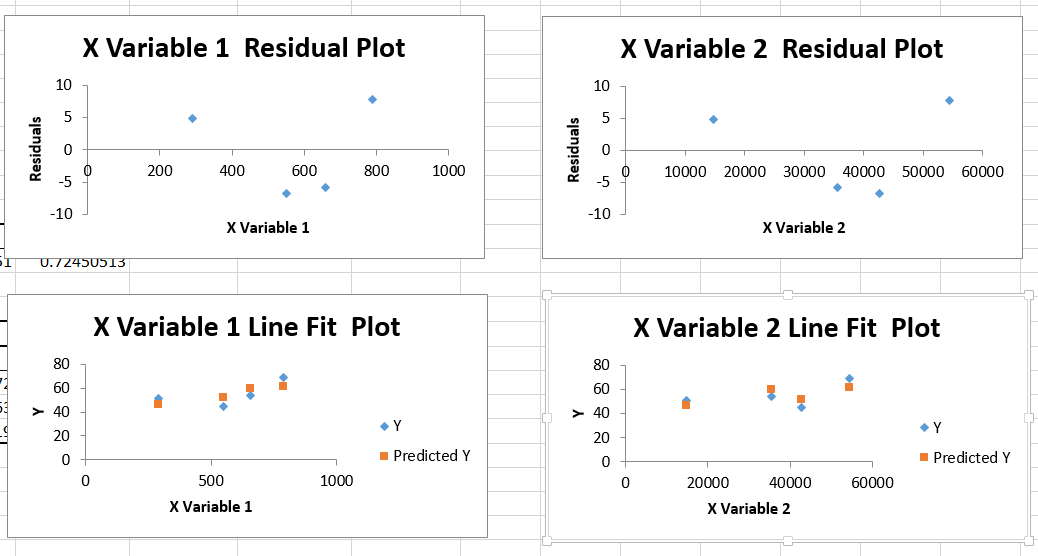# How to Do Regression Analysis in Excel

Regression analysis is a powerful statistical tool used to analyze and model the relationships between variables. It allows us to understand how changes in independent variables affect the value of a dependent variable. We will explore how to perform regression analysis in Microsoft Excel, specifically focusing on linear and multiple regression models.

## Accessing Regression Analysis in Microsoft Excel

Enable the Analysis ToolPak:

1. Click on File in the Excel ribbon.
2. Select Excel Options.
3. Click on Add-Ins in the left sidebar.
4. In the Manage box at the bottom, choose Excel Add-ins and click Go.Once you’ve enabled the Analysis ToolPak, you will have access to a range of data analysis tools, including regression.Data Analysis button will appear on Data ribbon. Under Data Analysis feature Regression function can be found.## Regression Analysis in Excel

Prepare X & Y values in the following style.Open Data Analysis and choose the Regression.

Fill the dialog box with ranges of your data:

• Input Y Range option: Select dependent variables,
• Input X Range option: Select independent variables,
• Output options specify how you would like the results to be displayed,
• Residuals contain options to draw the results as charts.Following regression table will be created in new sheet as follows.## Linear Regression in Excel

The linear regression function answers the question: “What value will a given phenomenon (dependent variable) take, depending on the value of another phenomenon (explanatory variable)?” Due to the term “linear”, a method which has a way to remove the explained phenomenon and the variable explanation is precisely linear.

To run the linear regression prepare your data.Select the cells for y-values, then the ones for x-values. Check the labels.Insert a scatter chart.Right-click on the chart and choose Select Data.Write the Series Name, choose the cells for X-values, and for Y-values.Click Ok.Right-click on any of the markers and select Add Trendline.Check the Display Equation on Chart and the R-square value on chart.You should have done a linear regression that looks like this:## Multiple Regression in Excel

Multiple regression extends linear regression by considering the relationship between a dependent variable and multiple independent variables. Here’s how to perform multiple regression in Excel:

The first thing is having a data that could look something like this:Choose the data you want to analyze on X values, and Y values. Select desired options, under the Residuals.The multiple regression would look something like this:##Interpreting the Results

When interpreting the results of a regression analysis in Excel, consider the following:

### Regression Statistics

• Multiple R (Correlation Coefficient): A value higher than 0 indicates a positive relationship, with a higher number signifying a stronger relationship.
• R-squared (Coefficient of Determination): Indicates the proportion of values that fit the model, with higher values indicating a better fit.
• Adjusted R-squared: Particularly useful when you have multiple independent variables.
• Standard Error: A high standard error suggests less confidence in the analysis.
• Observations: The number of data points used in the analysis.

### Residual Output

• Predicted Y: Values predicted by the model.
• Residuals: Measure how far actual data points deviate from predicted values.

To enhance the accuracy of your analysis, aim to minimize residual values.